# Calculate Min & Max by Group in R (4 Examples)

In this article you’ll learn how to get the minimum and maximum by groups in a data frame in the R programming language.

The tutorial consists of four examples for the calculation of the minimum and maximum values by groups in a data frame. To be more specific, the content looks as follows:

Sound good? Let’s dive right in:

## Construction of Example Data

We use the following data as a basement for this R tutorial:

```data <- data.frame(value = 1:9,                             # Create example data frame
group = rep(LETTERS[1:3], each = 3))
data                                                        # Print example data frame```Table 1 shows the structure of our example data – It consists of nine rows and two variables.

## Example 1: Calculate Min & Max by Group Using Base R

This example explains how to use the basic installation of the R programming language to calculate minimum and maximum values by group.

To accomplish this, we can use the aggregate function. Let’s first calculate the minima by group:

```data_min1 <- aggregate(formula = value ~ group,             # Get min by group
data = data,
FUN = min)
data_min1                                                   # Return min by group```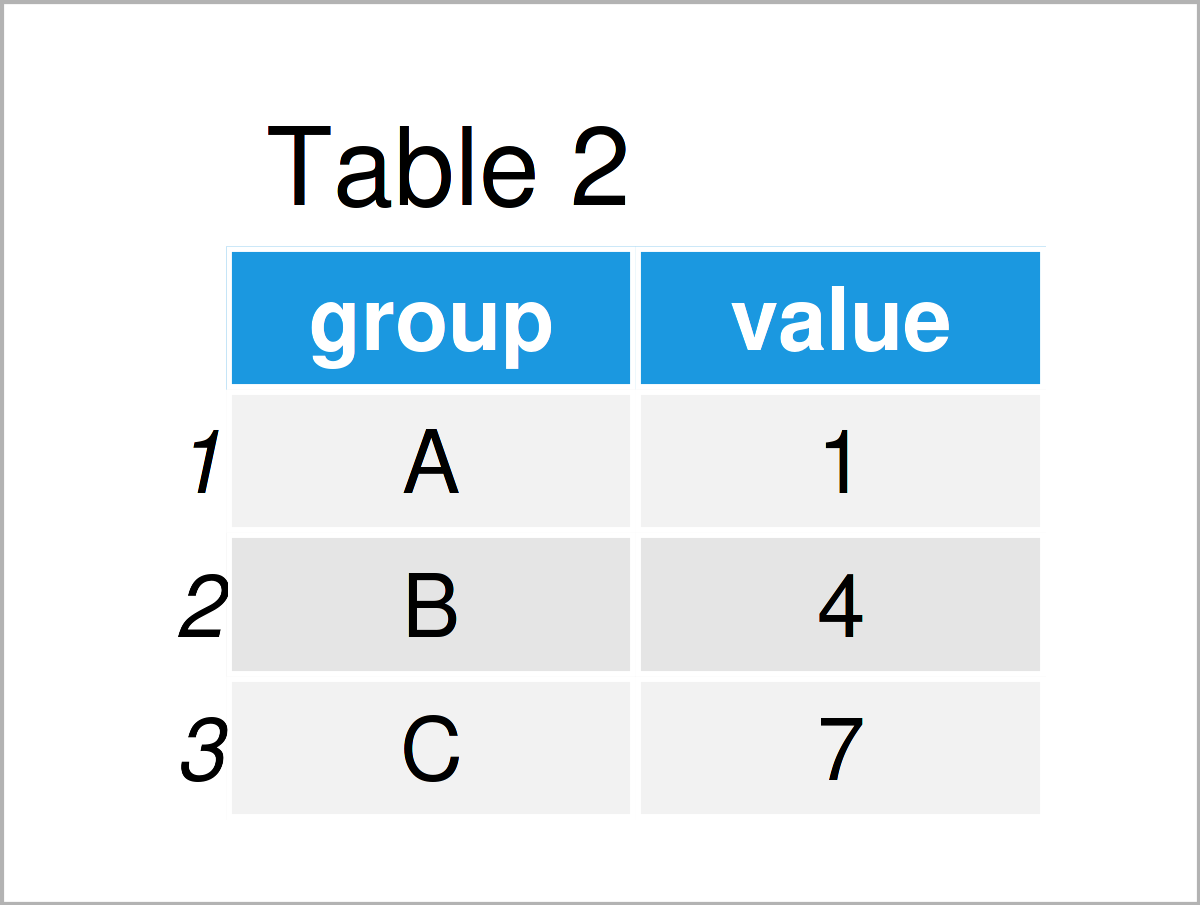By executing the previous R code, we have created Table 2, i.e. a data set containing the minimum value of each group.

Similar to that, we can also use the aggregate function to return the maximum value for each group:

```data_max1 <- aggregate(formula = value ~ group,             # Get max by group
data = data,
FUN = max)
data_max1                                                   # Return max by group```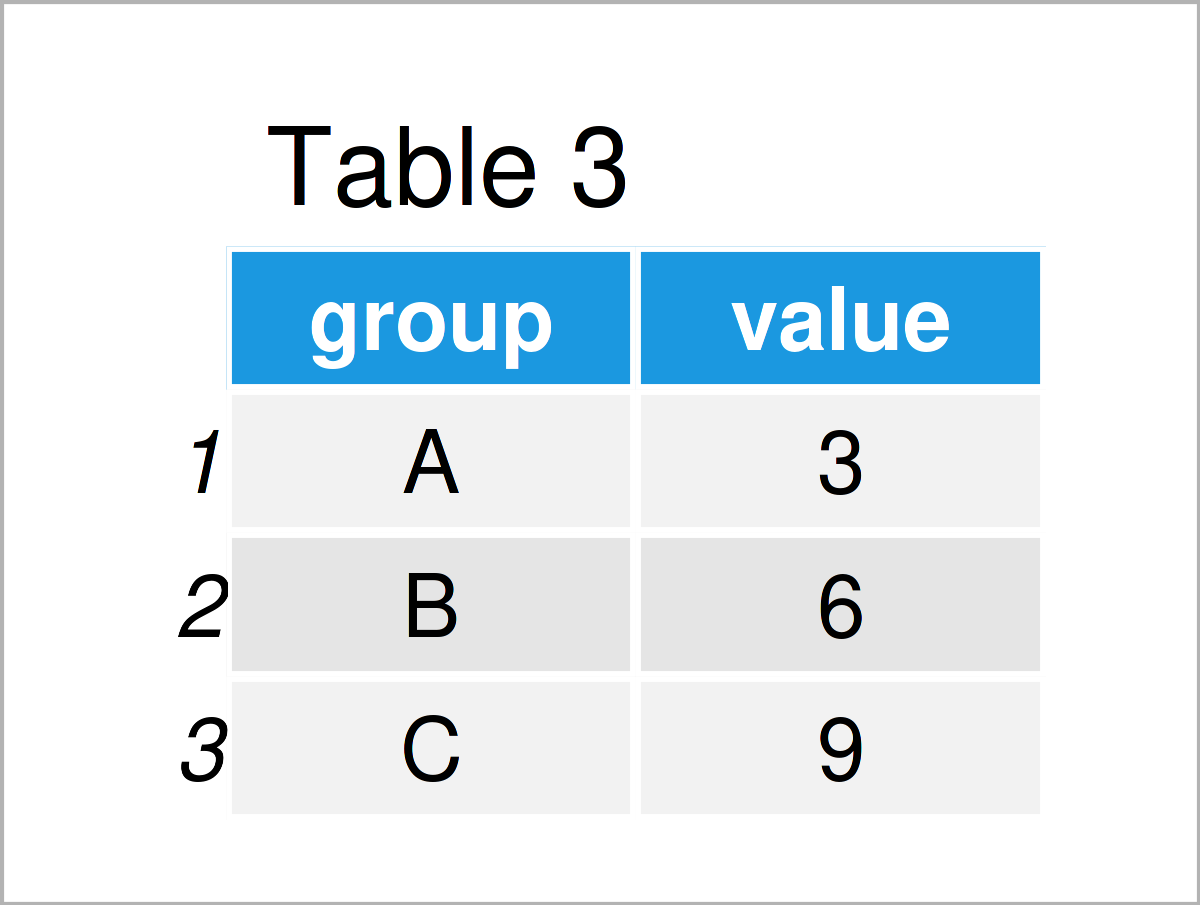Table 3 shows the output of the previous R programming syntax – The maxima by groups in our data frame.

## Example 2: Calculate Min & Max by Group Using dplyr Package

Example 1 has explained how to compute the min and max values by group using base R.

In this example, I’ll illustrate how to apply the functions of the dplyr package to achieve this.

If we want to use the functions of the dplyr package, we first need to install and load dplyr to R:

```install.packages("dplyr")                                   # Install & load dplyr
library("dplyr")```

In the next step, we can use the group_by and summarise_at functions to return the minimum value by group:

```data_min2 <- data %>%                                       # Get min by group
group_by(group) %>%
summarise_at(vars(value),
list(min = min))
data_min2                                                   # Return min by group```By exchanging the min by the max function, we can return the maximum value by group as well:

```data_max2 <- data %>%                                       # Get max by group
group_by(group) %>%
summarise_at(vars(value),
list(max = max))
data_max2                                                   # Return max by group```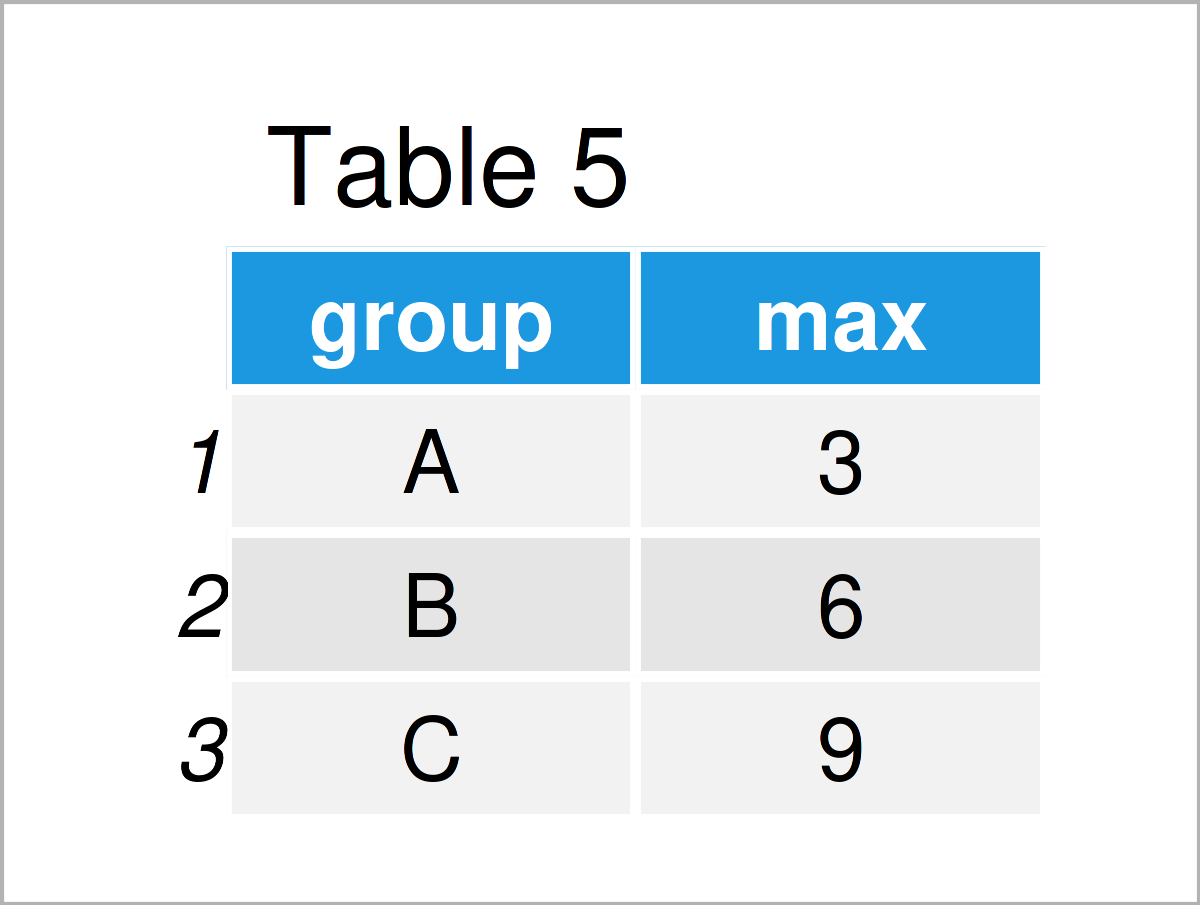As you can see, the output values of the previous R syntax are the same as in Example 1. Note that those outputs are stored in tibbles instead of data.frames.

## Example 3: Calculate Min & Max by Group Using data.table Package

In Example 3, I’ll demonstrate how to use the data.table package to calculate minimum and maximum vales by group.

We first need to install and load the data.table package, if we want to use the functions that are contained in the package:

```install.packages("data.table")                              # Install & load data.table package
library("data.table")```

Now, we can apply the following R code to get the minimum values by group:

```data_min3 <- data.table(data)                               # Convert data.frame to data.table
data_min3 <- data_min3[ , .(min = min(value)), by = group]  # Get min by group
data_min3                                                   # Return min by group```Similar to the previous code, we may use the syntax below to print the maxima by group:

```data_max3 <- data.table(data)                               # Convert data.frame to data.table
data_max3 <- data_max3[ , .(max = max(value)), by = group]  # Get max by group
data_max3                                                   # Return max by group```Once again, we have returned the same output values. This time our output is stored in data.tables.

## Example 4: Calculate Min & Max by Group & Add as a New Column

The following code illustrates how to add minimum and maximum values by group as a new column to a data frame object.

To accomplish this, we can use the ave function as shown below:

```data_min4 <- data                                           # Replicate data frame
data_min4\$min <- ave(data_min4\$val,                         # Get min by group
data_min4\$gr,
FUN = min)
data_min4                                                   # Return min by group as column```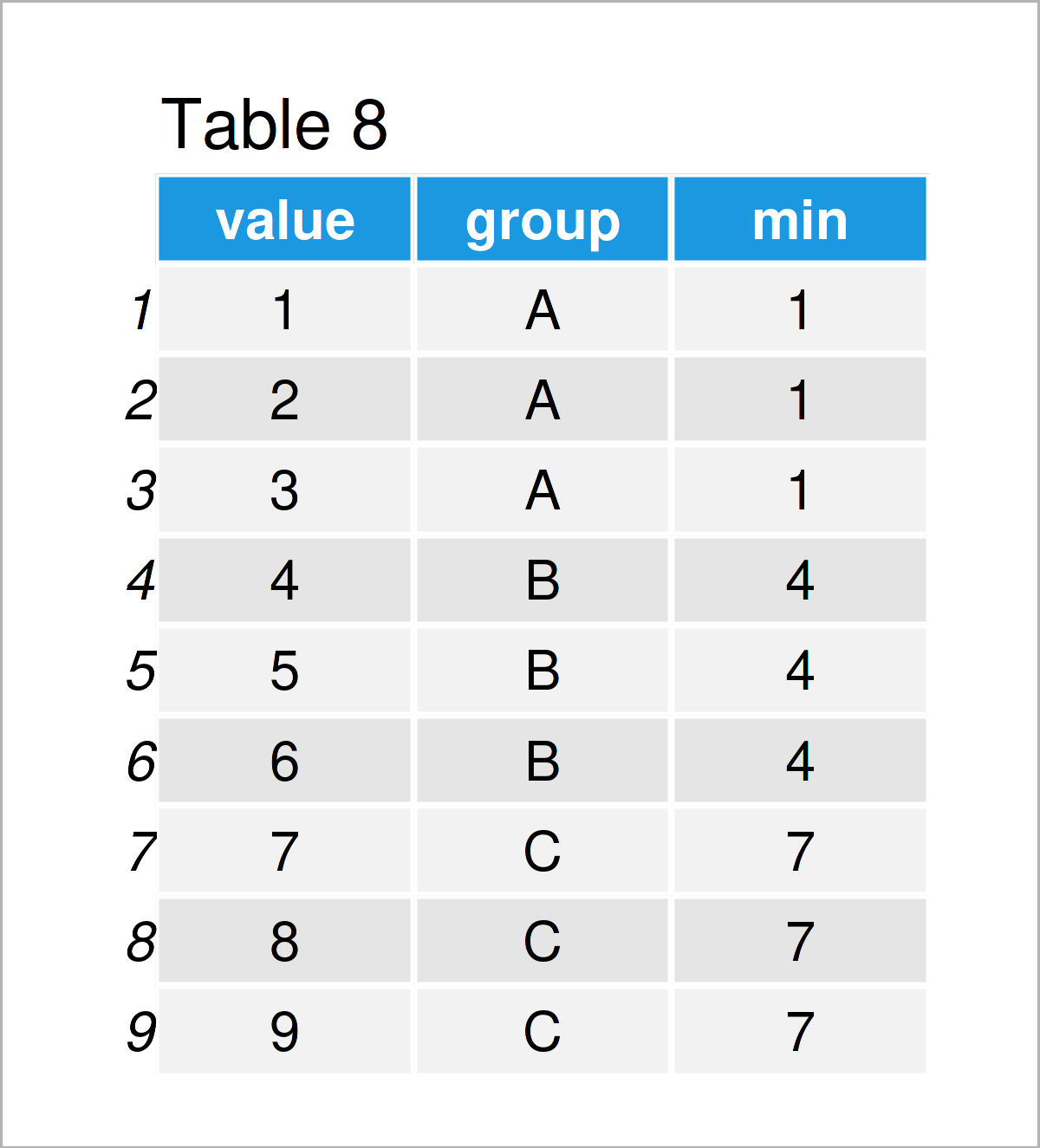After running the previous R code the data frame shown in Table 8 has been created. As you can see, we have added a new variable to our input data frame, which contains the min values by group that correspond to each row.

We also may use the ave function to get the maximum value by group as a new column:

```data_max4 <- data                                           # Replicate data frame
data_max4\$max <- ave(data_max4\$val,                         # Get max by group
data_max4\$gr,
FUN = max)
data_max4                                                   # Return max by group as column```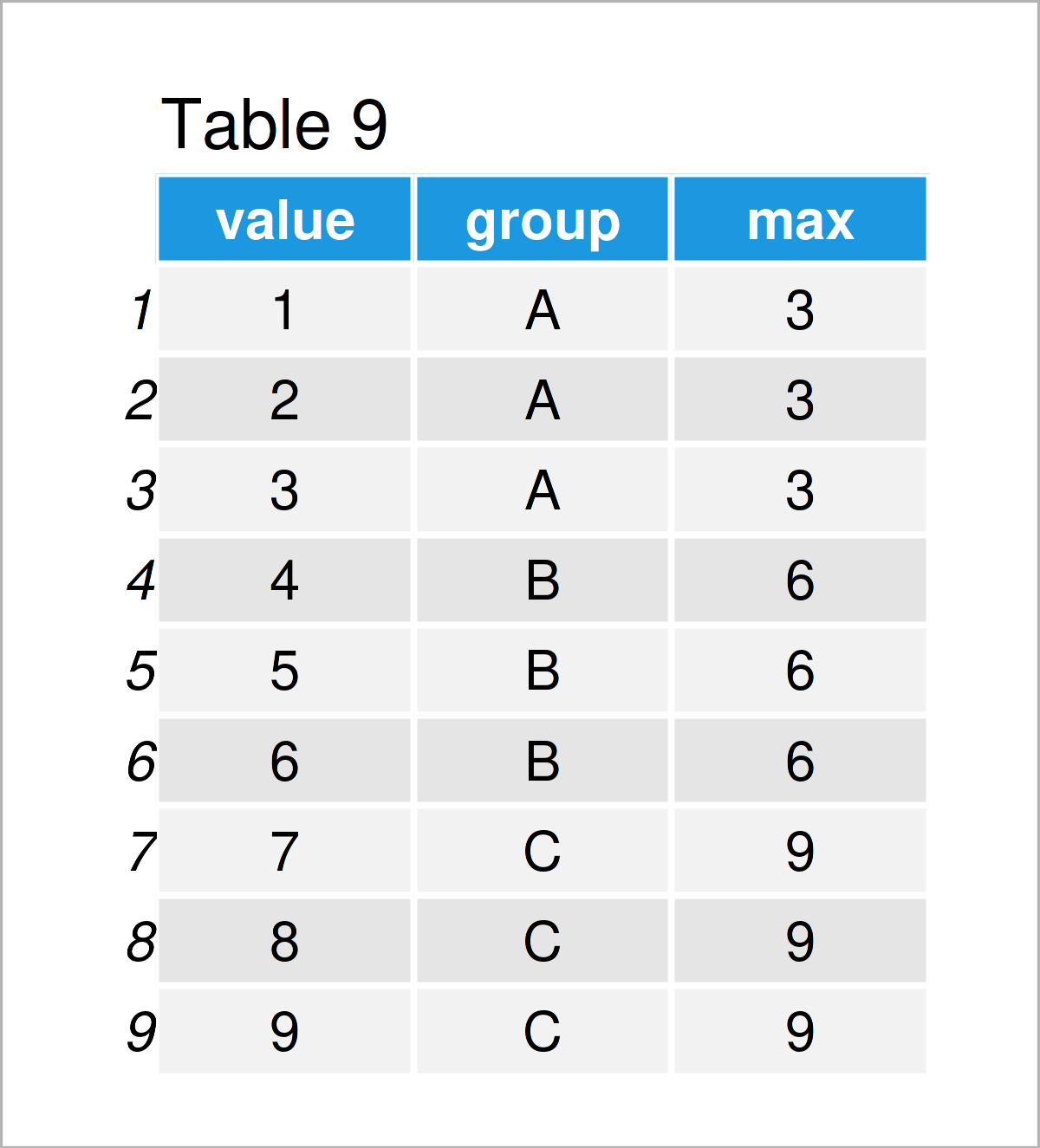In case you want to learn more on how to append the maximum value by group as a new column to a data frame, you may have a look here.

## Video & Further Resources

Do you need more info on the topics of the present tutorial? Then you might have a look at the following video instruction on my YouTube channel. I’m explaining the R code of this article in the video.

In addition, you may want to have a look at some of the other articles on my website.

In summary: This tutorial has explained how to calculate the minimum and maximum by group in the R programming language. Don’t hesitate to let me know in the comments, if you have additional questions.

Subscribe to the Statistics Globe Newsletter